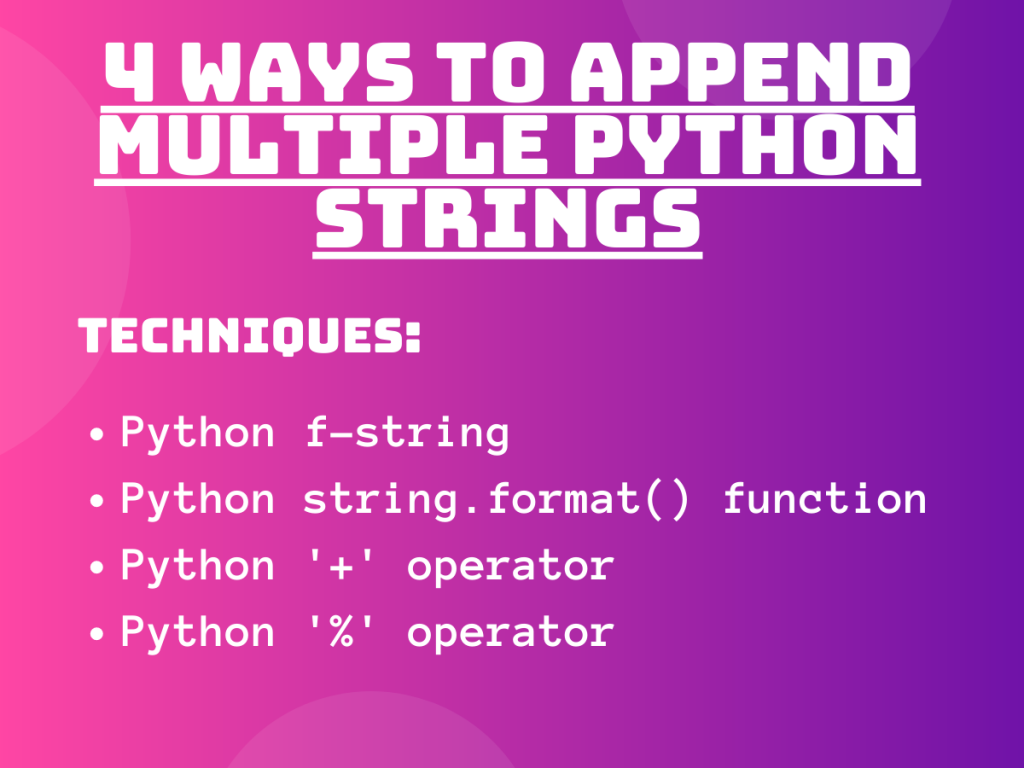# 4 Easy Methods to Append Multiple Strings in PythonIn this article we will be having a look at the different ways to interpolate and append multiple strings in Python. String interpolation involves an injection of string in a particular statement. Let’s get right into it!

## Technique 1: f-string to append multiple strings in Python

Python f-string is also known as format-strings have proved to be an efficient and optimal way to deal with strings. The f-string was introduced under the PEP 498 as Literal String Interpolation.

The `f-string` basically serves the purpose of `string interpolation` i.e. injection of multiple strings into a statement or structure.

Syntax:

```f'{string1} {string2} {stringN}'
```
• {}: The string to be interpolated is placed between the curly braces.

Example 1:

```str1 = 'Python'
str2 = '@'
str3 = 'JournalDev'
res = f'{str1} {str2} {str3}'
print("Appending multiple strings using f-string:\n")
print(res)
```

Output:

```Appending multiple strings using f-string:

Python @ JournalDev
```

Example 2:

```str1 = 'Python'
str2 = 'and'
str3 = 'R'
str4 = '@ JournalDev'
res = f'{str1} {str2} {str3} {str4}'
print("Appending multiple strings using f-string:\n")
print(res)

```

Output:

```Appending multiple strings using f-string:

Python and R @ JournalDev
```

## Technique 2: Python format() method to append multiple strings

Python string.format() function can also be used to format strings efficiently.

Syntax:

1. Single string formatting using format() function

```{}.format(string)
```

2. Multiple string formatting using format() function

```{} {}.format(string1, string2)
```

The `string.format() function` formats the strings and helps in the substitution of string through positional formatting i.e. according to the position of string placed in the function parameter list.

Example 1:

```str1 = 'Python'
str2 = '@'
str3 = 'JournalDev'
res = "{} {} {}".format(str1, str2, str3)
print("Appending multiple strings using format():\n")
print(res)

```

Output:

```Appending multiple strings using format():

Python @ JournalDev
```

Example 2:

```str1 = 'Python'
str2 = 'and'
str3 = 'Java'
str4 = '@ JournalDev'
res = "{} {} {} {}".format(str1, str2, str3, str4)
print("Appending multiple strings using format():\n")
print(res)

```

Output:

```Appending multiple strings using format():

Python and Java @ JournalDev
```

Let’s learn some more ways to append multiple strings in Python.

## Technique 3: Using ‘+’ operator to append multiple strings

Python concatenation operator i.e. `'+' operator` can be used to append multiple strings together.

Syntax:

```string1 + string2 + ..... + stringN
```

Example 1:

```str1 = 'Python'
str2 = '@'
str3 = 'JournalDev'

res = str1 + str2 + str3
print("Appending multiple strings using Python '+' operator:\n")
print(res)

```

Output:

```Appending multiple strings using Python '+' operator:

[email protected]
```

Example 2:

```str1 = 'Python'
str2 = '+'
str3 = 'R'
str4 = "@JournalDev"

res = str1 + str2 + str3 + str4
print("Appending multiple strings using Python '+' operator:\n")
print(res)

```

Output:

```Appending multiple strings using Python '+' operator:

[email protected]
```

## Technique 4: Python ‘%’ operator to append multiple strings

Python `'%' operator` serves the purpose of string formatting and interpolation.

Syntax:

```"%s" % (string)
```

The ‘%s’ serves as a placeholder to replace it with the string passed in the parenthesis ().

Example 1:

```str1 = 'Python'
str2 = '+'
str3 = 'Java'

res = "%s %s %s" % (str1, str2, str3)
print("Appending multiple strings using Python '%' operator:\n")
print(res)

```

Output:

```Appending multiple strings using Python '%' operator:

Python + Java
```

Example 2:

```str1 = 'Python'
str2 = '+'
str3 = 'Java'
str4 = '@ journalDev'

res = "%s %s %s %s" % (str1, str2, str3, str4)
print("Appending multiple strings using Python '%' operator:\n")
print(res)
```

Output:

```Appending multiple strings using Python '%' operator:

Python + Java @ journalDev
```

## Conclusion

Thus, in this article, we have understood different ways to interpolate and append multiple strings in Python.

## References

• Python String concatenation – JournalDev